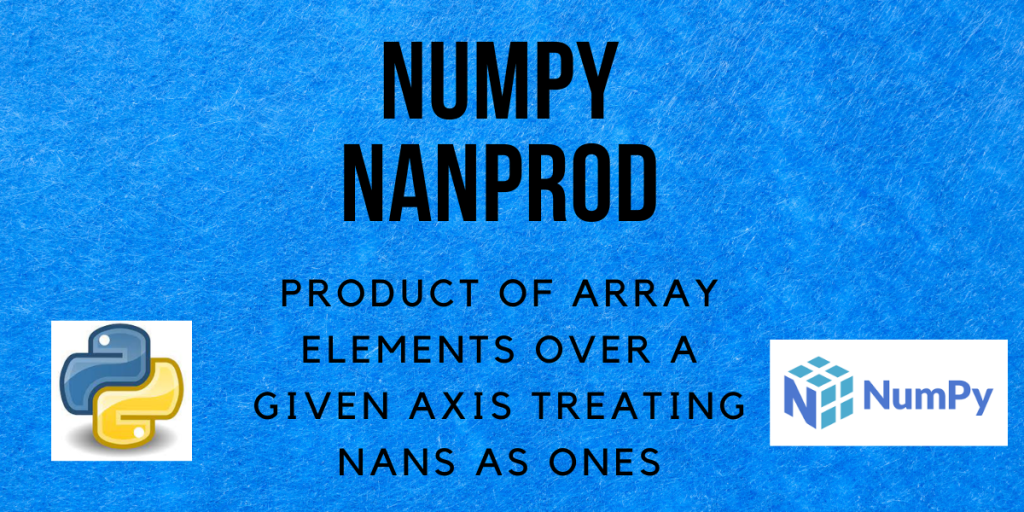# NumPy nanprod – A Complete GuideHello and welcome to this tutorial on Numpy nanprod. In this tutorial, we will be learning about the NumPy nanprod() method and also seeing a lot of examples regarding the same. So let us begin!

Also read: Numpy trunc() – Return the truncated value of the input, element-wise

## What is NumPy nanprod?

In Python, NaN denotes Not a Number. If we have an array that contains some NaN values and want to find its product, we can use the `nanprod()` method from NumPy.

The `nanprod()` method in NumPy is a function that returns the product of the array elements calculated by treating the NaN values in the array as equal to 1. It can be the product of all the array elements, the product of the array elements along the rows or the product of the array elements along the columns.

We will see the examples for each of these in the upcoming section of this tutorial.

## Syntax of NumPy nanprod

```numpy.nanprod(a, axis=None, dtype=None, out=None, keepdims=<no value>, initial=<no value>, where=<no value>)
```

Returns:
An array with the same shape as a which contains the product of the elements of a by treating NaN values as 1, along the axis given and the specified axis removed. If the axis=None, a scalar is returned which is the product of the whole array.

## Examples of numpy.nanprod()

The `numpy.nanprod()` function is used to compute the product of array elements over a given axis, ignoring NaNs. Let’s take a look at the usage of `numpy.nanprod()` along with some examples.

### Product of the whole array using numpy.nanprod()

1-dimensional array

```import numpy as np

a = np.array([6, np.nan, 7])
product = np.nanprod(a)
print("a =", a)
print("Product of the array =", product)
```

Output:

```a = [ 6. nan  7.]
Product of the array = 42.0
```

In the above code, the array contains one NaN value. While calculating the product, the `nanprod()` function treats that NaN value as 1 and calculates the product as 6*1*7 = 42.

2-dimensional array

```import numpy as np

a = np.array([[6, np.nan, 7], [np.nan, np.nan, 3]])
product = np.nanprod(a)
print("a =", a)
print("Product of the array =", product)
```

Output:

```a = [[ 6. nan  7.]
[nan nan  3.]]
Product of the array = 126.0
```

Treating all the NaN values as 1, product = 6*1*7*1*1*3 = 126.

### Product along the axis

Column-wise product

```import numpy as np

a = np.array([[np.nan, np.nan, 4],
[5, np.nan, 10]])

# product along axis=0 i.e. columns
product = np.nanprod(a, axis=0)
print("a =", a)
print("Product of the array =", product)
```

Output:

```a = [[nan nan  4.]
[ 5. nan 10.]]
Product of the array = [ 5.  1. 40.]
```

Treating NaN values as 1,
Column 0 product = 1*5 = 5
Column 1 product= 1*1 = 1
Column 2 product = 4*10 = 40

Row-wise product

```import numpy as np

a = np.array([[np.nan, np.nan, 4],
[5, np.nan, 10]])

# product along axis=1 i.e. rows
product = np.nanprod(a, axis=1)
print("a =", a)
print("Product of the array =", product)
```

Output:

```a = [[nan nan  4.]
[ 5. nan 10.]]
Product of the array = [ 4. 50.]
```

Treating NaN values as 1,
Row 0 product = 1*1*4 = 4
Row 1 product = 5*1*10 = 50

## Product of an empty array and an all NaN array

```import numpy as np

# empty arrays
a = []
b = [[]]

product_a = np.nanprod(a)
print("a =", a)
print("Product of the 1-d empty array =", product_a)

product_b = np.nanprod(b)
print("b =", b)
print("Product of the 2-d empty array =", product_b)

# all NaN array
c = [np.nan, np.nan, np.nan]
product_c = np.nanprod(c)
print("c =", c)
print("Product of the all NaN array =", product_c)
```

Output:

```a = []
Product of the 1-d empty array = 1.0
b = [[]]
Product of the 2-d empty array = 1.0
c = [nan, nan, nan]
Product of the all NaN array = 1.0
```

All the empty arrays and the arrays containing only NaN values return 1 when the `nanprod()` method is applied to them.

## Conclusion

That’s all! In this tutorial, we learned about the Numpy nanprod method and practiced different types of examples using the same.